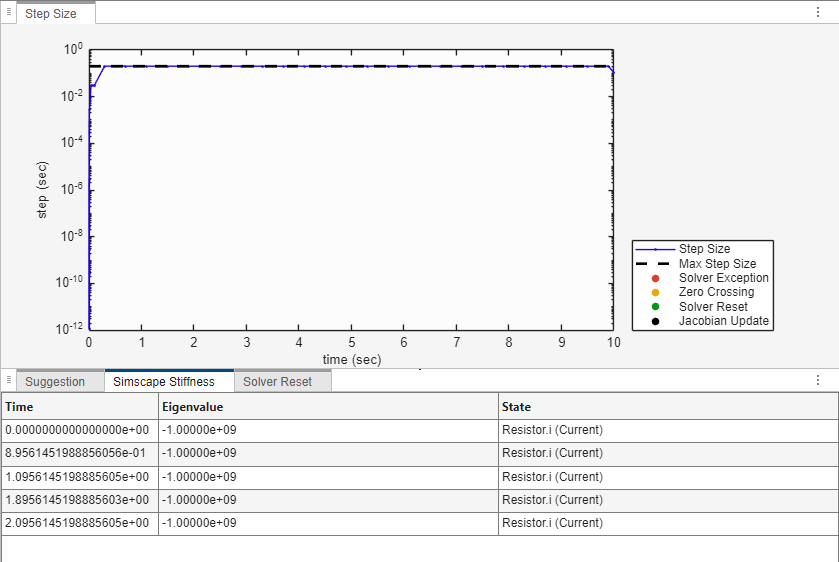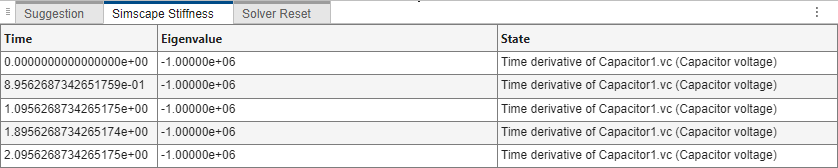## Simscape Stiffness Impact Analysis

When you use an explicit solver, the simulation may become unstable because the system is stiff. To learn more, see Explicit Versus Implicit Continuous Solvers. In most models, you can change the block parameter values to reduce system stiffness and remove the instability. The Stiffness Impact Analysis option in the Solver Profiler tool lets you analyze the Simscape™ networks in your model and determine which variables and equations have the most impact on system stiffness. You can then modify the values of parameters involved in these equations to make the system less stiff.

To perform impact analysis on your Simscape model, click the Simscape Stiffness drop-down button in the Solver Profiler interface, and enter the stiffness analysis checkpoints as a vector.

For example, consider a model where a resistor and capacitor are connected in series with a DC voltage source.To access the Stiffness Impact Analysis tool, open the Solver Profiler by clicking the hyperlink in the lower-right corner of the model window.Click the Simscape Stiffness drop down menu in the Solver Profiler toolstrip. Enter a vector, and click Run. This example uses the vector `[0, 1, 2]`.The Simscape Stiffness tab in the bottom pane shows that the variable with the most impact on the system stiffness is `i(Current)` in the Resistor block, and the corresponding eigenvalue is `-9.999999e+08` at each interval. Note that when the time steps do not line up with the times in your vector, the tool performs stiffness analysis at the closest time steps before and after the instances you specify, t- and t+, respectively.

Tip

The greater the magnitude of a negative eigenvalue, the more unstable the system becomes due to stiffness.The Resistor block uses the equation

`v == R*i;`

Note that the block derives the current from the resistance. To reduce the stiffness due to the current, reduce the value of the resistance. For example, if you set Resistance to `1e-6` `Ohm` and rerun the simulation using the Stiffness Impact Analysis tool, the corresponding eigenvalues drop to `-5.00000e+08`. A reduction of this magnitude may be enough for your model to run successfully.The Stiffness Impact Analysis tool has the following limitations:

• The tool performs stiffness analysis of the Simscape networks only. If a model does not contain Simscape blocks, the tool does not show any results.

• The tool does not produce results and issues a warning if the system is algebraic, high-index, uses frequency-and-time equation formulation, or contains Simscape Multibody™ blocks.

• The tool does not work with Simscape Electrical™ Specialized Power Systems.